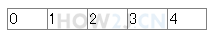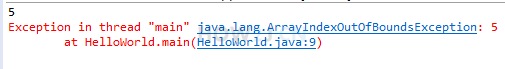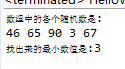8分35秒

## 声明数组

int[] a; 声明了一个数组变量。
[]表示该变量是一个数组
int 表示数组里的每一个元素都是一个整数
a 是变量名

public class HelloWorld { public static void main(String[] args) { // 声明一个数组 int[] a; } }
```public class HelloWorld {
public static void main(String[] args) {
// 声明一个数组
int[] a;
}
}
```

## 创建数组

new int

int c = 5; 这叫给c赋值为5

a = new int;public class HelloWorld { public static void main(String[] args) { //声明一个引用 int[] a; //创建一个长度是5的数组，并且使用引用a指向该数组 a = new int; int[] b = new int; //声明的同时，指向一个数组 } }
```public class HelloWorld {
public static void main(String[] args) {
//声明一个引用
int[] a;
//创建一个长度是5的数组，并且使用引用a指向该数组
a = new int;

int[] b = new int; //声明的同时，指向一个数组

}
}
```

## 访问数组public class HelloWorld { public static void main(String[] args) { int[] a; a = new int; a= 1; //下标0，代表数组里的第一个数 a= 2; a= 3; a= 4; a= 5; } }
```public class HelloWorld {
public static void main(String[] args) {
int[] a;
a = new int;

a= 1;  //下标0，代表数组里的第一个数
a= 2;
a= 3;
a= 4;
a= 5;
}
}
```

## 数组长度

.length属性用于访问一个数组的长度public class HelloWorld { public static void main(String[] args) { int[] a; a = new int; System.out.println(a.length); //打印数组的长度 a=100; //下标4，实质上是“第5个”，即最后一个 a=101; //下标5，实质上是“第6个”，超出范围 ,产生数组下标越界异常 } }
```public class HelloWorld {
public static void main(String[] args) {
int[] a;
a = new int;

System.out.println(a.length); //打印数组的长度

a=100; //下标4，实质上是“第5个”，即最后一个
a=101; //下标5，实质上是“第6个”，超出范围 ,产生数组下标越界异常

}
}
```

## 练习-数组最小值

姿势不对,事倍功半! 点击查看做练习的正确姿势

0-100的 随机整数的获取办法有多种，下面是参考办法之一:

(int) (Math.random() * 100)

Math.random() 会得到一个0-1之间的随机浮点数，然后乘以100，并强转为整型即可。
public class HelloWorld { public static void main(String[] args) { int[] a = new int; a = (int) (Math.random() * 100); a = (int) (Math.random() * 100); a = (int) (Math.random() * 100); a = (int) (Math.random() * 100); a = (int) (Math.random() * 100); System.out.println("数组中的各个随机数是:"); for (int i = 0; i < a.length; i++) System.out.println(a[i]); System.out.println("本练习的目的是，找出最小的一个值: "); } }
```public class HelloWorld {
public static void main(String[] args) {
int[] a = new int;
a = (int) (Math.random() * 100);
a = (int) (Math.random() * 100);
a = (int) (Math.random() * 100);
a = (int) (Math.random() * 100);
a = (int) (Math.random() * 100);

System.out.println("数组中的各个随机数是:");
for (int i = 0; i < a.length; i++)
System.out.println(a[i]);

System.out.println("本练习的目的是，找出最小的一个值: ");
}
}
```

## 答案-数组最小值3分49秒 本视频采用html5方式播放，如无法正常播放，请将浏览器升级至最新版本，推荐火狐，chrome，360浏览器。 如果装有迅雷，播放视频呈现直接下载状态，请调整 迅雷系统设置-基本设置-启动-监视全部浏览器 (去掉这个选项)。 chrome 的 视频下载插件会影响播放，如 IDM 等，请关闭或者切换其他浏览器public class HelloWorld { public static void main(String[] args) { int[] a = new int; a = (int) (Math.random() * 100); a = (int) (Math.random() * 100); a = (int) (Math.random() * 100); a = (int) (Math.random() * 100); a = (int) (Math.random() * 100); System.out.println("数组中的各个随机数是:"); for (int i = 0; i < a.length; i++) System.out.print(a[i] + " "); System.out.println(); int min = 100; for (int i = 0; i < a.length; i++) { if( a[i] < min ) min = a[i]; } System.out.println("找出来的最小数值是:" +min); } }

HOW2J公众号，关注后实时获知最新的教程和优惠活动，谢谢。2023-03-19 分享一道有点难度的题，是翻以前的评论看到有兄弟分享的，大家试着做一做
 15032CXJ 关于 JAVA 基础-数组-创建数组 的提问 1，给大家分享一道题，是我翻以前的评论，有位兄弟分享的，有一定难度，我也跟着做了一遍，觉得不错，故分享出来，大家试着做一做 2，题目如下： ①利用Math.random方法创建二维数组a和二维数组所包含的一维数组a1、a2、a3。。。（话说的比较绕，大家先查查多维数组的概念，二维数组的组成元素是一维数组） ②打印该二维数组 ③把二维数组的内容复制给一个新创建的一维数组 ④打印该一维数组 ⑤对该一维数组进行排序（从小到大） ⑥打印排序后的一维数组 ⑦将排序后的一维数组的元素，赋给原二维数组 ⑧打印该二维数组 （那位兄弟也没说题目，这是我自己结合他的代码写的题目要求，大家凑合看） 3，注意点 ①题目比较综合，涉及到的知识点不少，有些目前的阶段可能大家还没学，做不出来就先放一放，等学的东西多了再回头试试， 大致有：1，二维数组，2，数组的排序，3，Math.random方法，4，冒泡排序法，5，单层、多层循环结构，6，数组的创建与声明 ，7， ②由于题目说的可能不大清楚，所以大家先把我的代码复制到软件上跑一遍，看看执行结果，不然的话容易懵逼。。。 ③这位兄弟用的一些知识我目前也没有学，所以我是在力所能及的情况下，用自己掌握的知识做出了这道题，大家在做的时候，也应该如此，他的代码中看不懂的，没学过的，就先不要用，用你知道的方法，先确保做出这道题，代码的优化以后再考虑 ④学习每一小节（每一节内容网页的最底下都有个按钮，点击能看所有的评论，不然只能看最近这段时间的评论，这些评论太少了，不够！！！，尽可能把以前的评论也看一遍，会很有收获的!！！）```//一，这是那位兄弟的代码，我的代码在下面 public static void main(String[] args){ //创建数组并填充随机数 int[][] a=new int[(int) (Math.random()*100)/10][]; for(int i=0;i b[k]) { int temp = b[k-1]; b[k-1] = b[k]; b[k] = temp; } } } //打印经过排序后的一维数组 System.out.println("========分割线======="); System.out.println("打印经过排序后的一维数组"); System.out.println("["); ; for (int i = 0; i < b.length; i++) { System.out.print(b[i] + " "); } System.out.println("]"); //将排序的一维数组填充到原二维数组，其实就是用排序后的二维数组替换原来的二维数组 int count2 = 0; for (int k = 0; k < a.length; k++) { for (int l = 0; l < a[k].length; l++) { a[k][l] = b[count2]; count2++; } } //打印排序后的二维数组 System.out.println("========分割线======="); System.out.println("打印排序后二维数组"); for (int i = 0; i < a.length; i++) { System.out.print("["); for (int m = 0; m < a[i].length; m++) { System.out.print(" " + a[i][m] + " "); } System.out.print("]"); System.out.println(); } }```

2 个答案

15032CXJ

15032CXJ

2023-02-17 简单一点
 云帆早日上岸 关于 JAVA 基础-数组-创建数组 的提问 康康我的 ```package priv.sun.array; public class ArrayCreate { public static void main(String[] args) { // 声明一个引用 int a[]; // 创建一个长度是5的数组，并且使用引用a指向该数组 a = new int; int min=200, temp=0; // 初始化min和temp for(int i=0;i

6 个答案

Closen1

public static void main(String[] args) { int[] a; a = new int; a=(int)(Math.random()*100); a=(int)(Math.random()*100); a=(int)(Math.random()*100); a=(int)(Math.random()*100); a=(int)(Math.random()*100); System.out.println("数组中各个随机数是："); int min=100,j=0; for (int i = 0; i < a.length; i++) { System.out.println("a["+i+"]"+"="+a[i]); } for (int i = 0; i <a.length; i++) { if (a[i]<min) { j = i; min = a[j]; } } System.out.println("随机数的最小数为:a["+j+"] = "+a[j]); }

2023-02-03 练习5 - 数组最小值
2023-01-06 求指点
2022-09-15 这个随机数调用挺方便的